# Electric Fields and Potential Electric Fields Every electric

• Slides: 13Electric Fields and Potential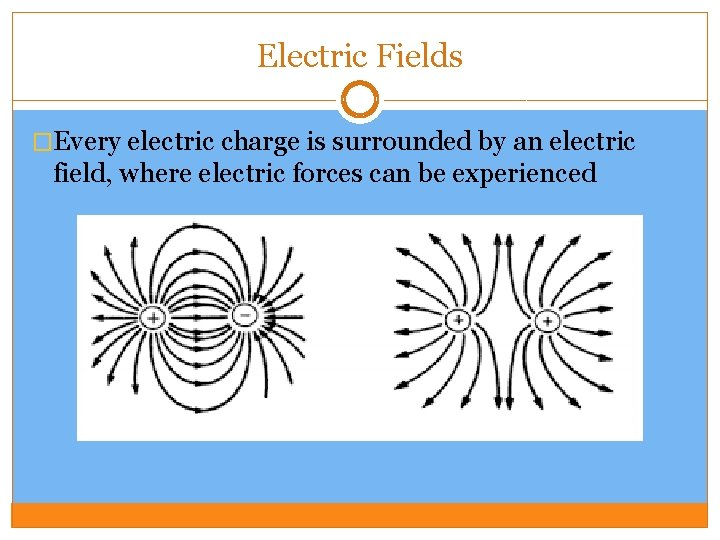Electric Fields �Every electric charge is surrounded by an electric field, where electric forces can be experienced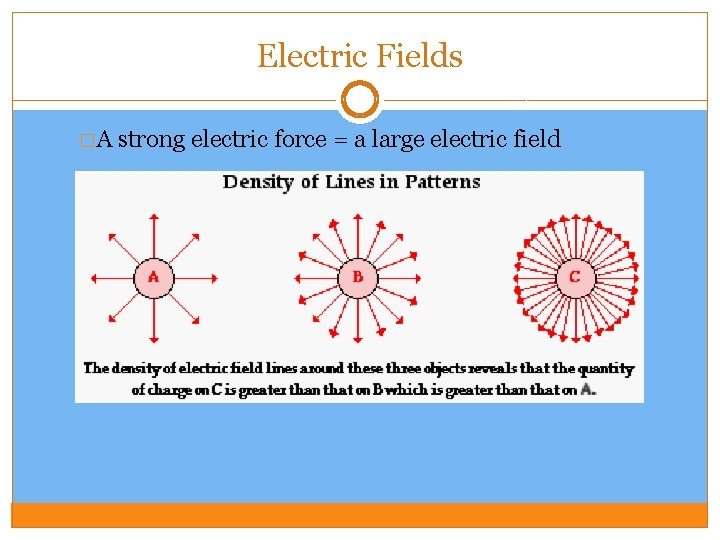Electric Fields �A strong electric force = a large electric field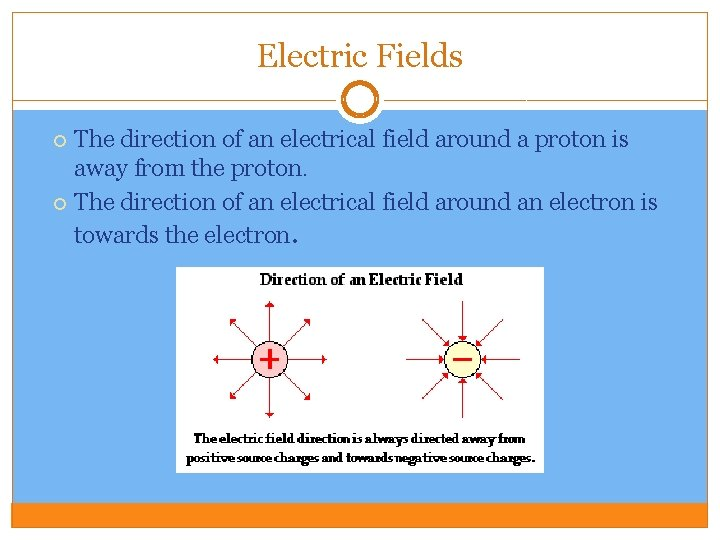Electric Fields The direction of an electrical field around a proton is away from the proton. The direction of an electrical field around an electron is towards the electron.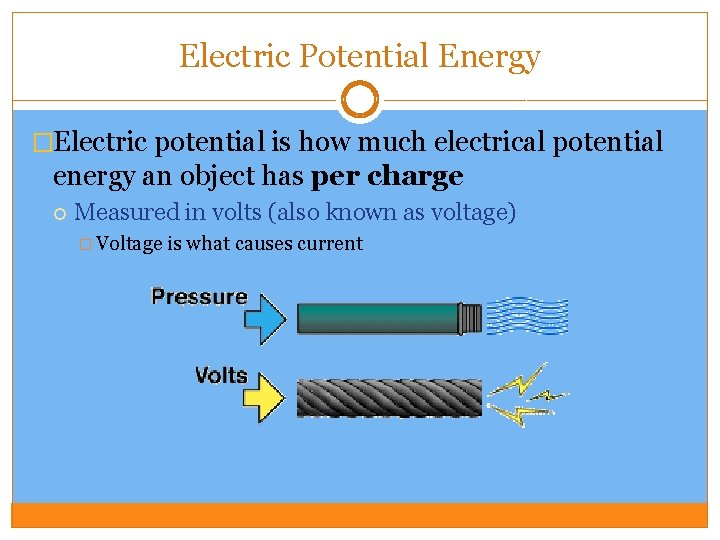Electric Potential Energy �Electric potential is how much electrical potential energy an object has per charge Measured in volts (also known as voltage) � Voltage is what causes current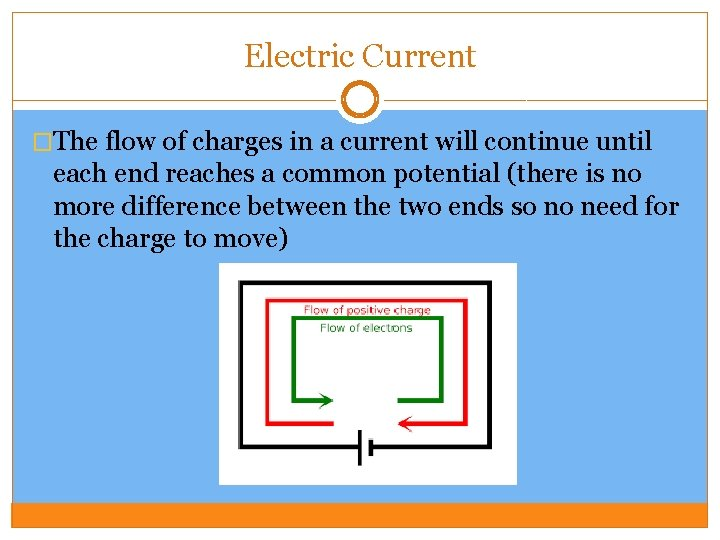Electric Current �The flow of charges in a current will continue until each end reaches a common potential (there is no more difference between the two ends so no need for the charge to move)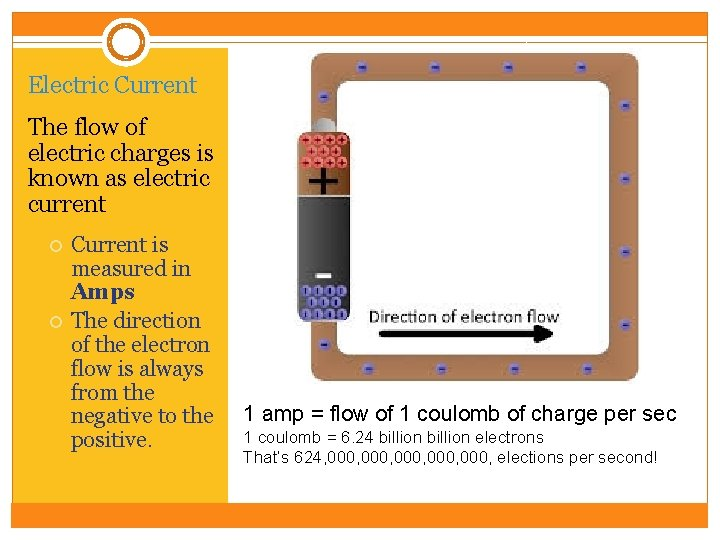Electric Current The flow of electric charges is known as electric current Current is measured in Amps The direction of the electron flow is always from the negative to the positive. 1 amp = flow of 1 coulomb of charge per sec 1 coulomb = 6. 24 billion electrons That’s 624, 000, 000, elections per second!Electric Current �To keep current flowing, there must be a constant potential difference in the conductor. �This is created using a voltage source Cells (batteries) � Convert Generators � Convert chemical energy into electrical energy mechanical energy into electric energy The voltage source provides “electric pressure” to move electrons through the conductor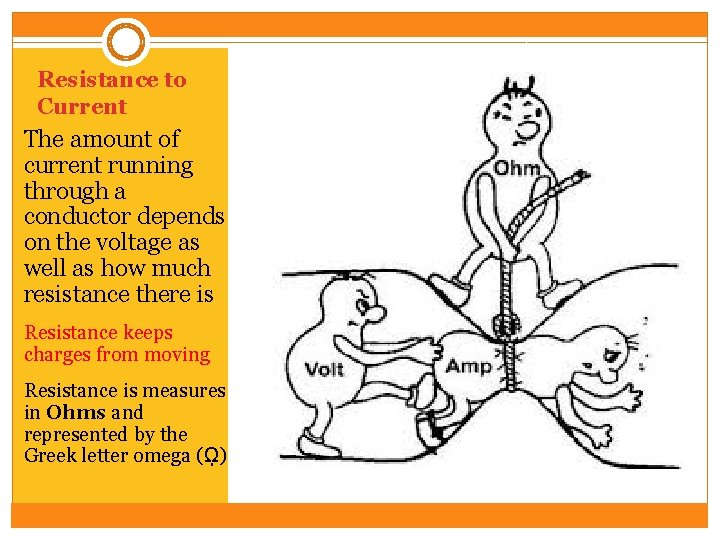Resistance to Current The amount of current running through a conductor depends on the voltage as well as how much resistance there is Resistance keeps charges from moving Resistance is measures in Ohms and represented by the Greek letter omega (ῼ)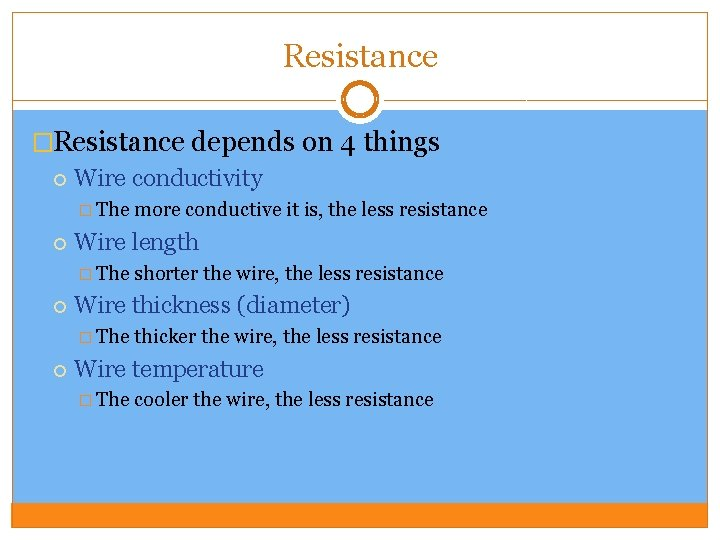Resistance �Resistance depends on 4 things Wire conductivity � The Wire length � The shorter the wire, the less resistance Wire thickness (diameter) � The more conductive it is, the less resistance thicker the wire, the less resistance Wire temperature � The cooler the wire, the less resistanceElectric Circuit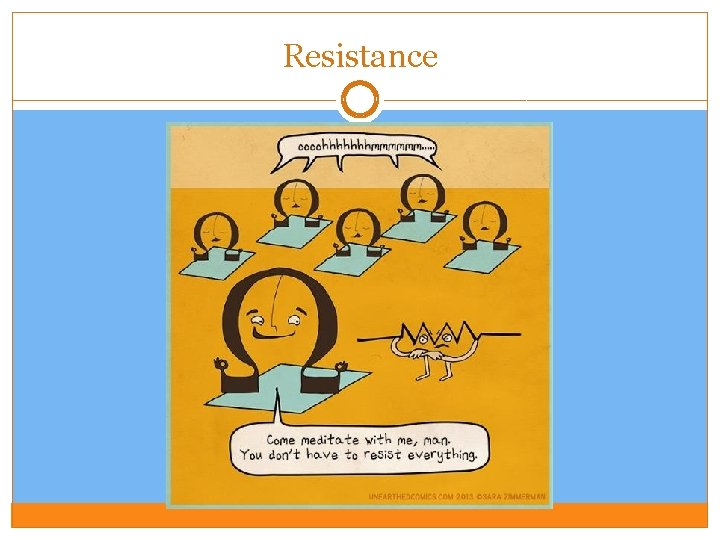Resistance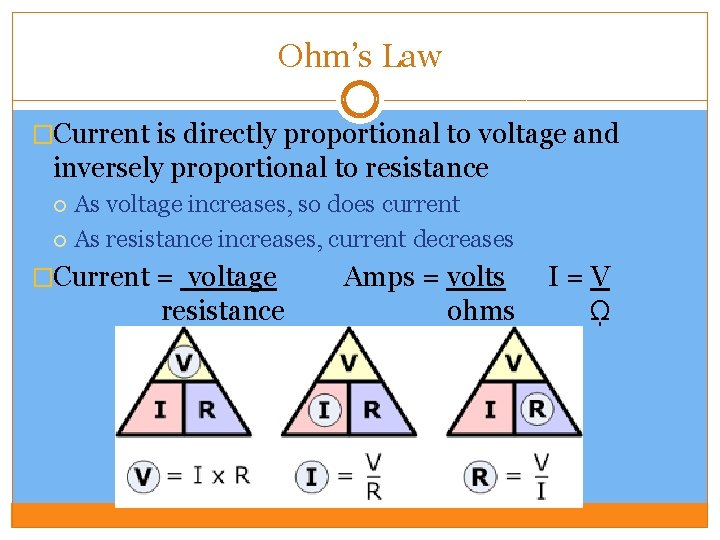Ohm’s Law �Current is directly proportional to voltage and inversely proportional to resistance As voltage increases, so does current As resistance increases, current decreases �Current = voltage resistance Amps = volts ohms I=V ῼ